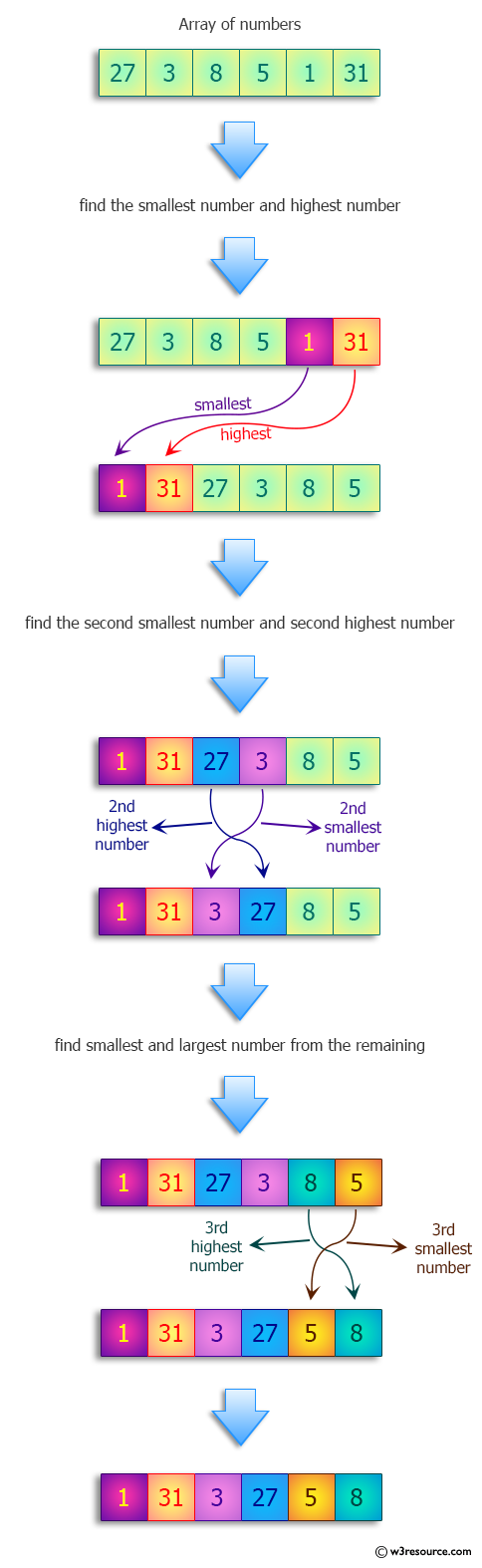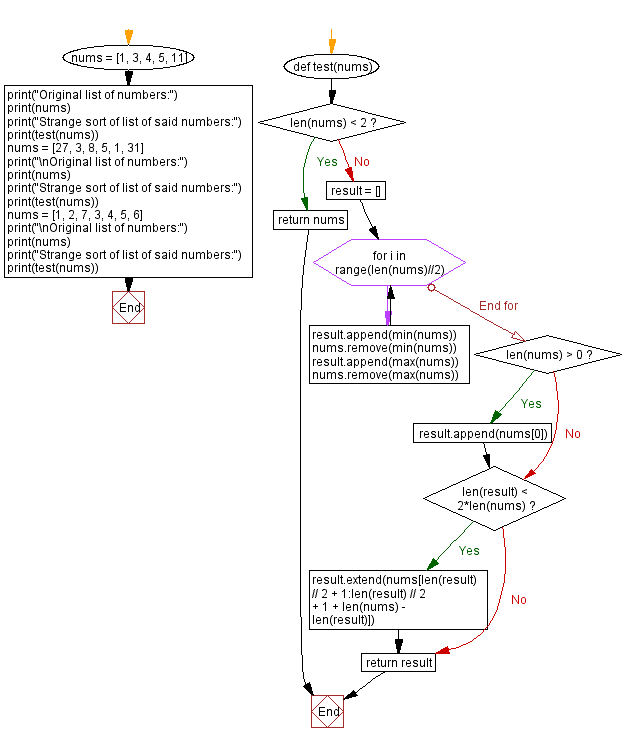﻿ Python: Strange sort of list of numbers - w3resource# Python: Strange sort of list of numbers

## Python Programming Puzzles: Exercise-97 with Solution

Write a Python program to find the following strange sort of list of numbers: the first element is the smallest, the second is the largest of the remaining, the third is the smallest of the remaining, the fourth is the smallest of the remaining, etc.

```Input:
[1, 3, 4, 5, 11]
Output:
[1, 11, 3, 5, 4]

Input:
[27, 3, 8, 5, 1, 31]
Output:
[1, 31, 3, 27, 5, 8]

Input:
[1, 2, 7, 3, 4, 5, 6]
Output:
[1, 7, 2, 6, 3, 5, 4]
```

Pictorial Presentation:Sample Solution:

Python Code:

``````#License: https://bit.ly/3oLErEI

def test(nums):
if len(nums) < 2:
return nums
result = []
for i in range(len(nums)//2):
result.append(min(nums))
nums.remove(min(nums))
result.append(max(nums))
nums.remove(max(nums))
if len(nums) > 0:
result.append(nums)
if len(result) < 2*len(nums):
result.extend(nums[len(result) // 2 + 1:len(result) // 2 + 1 + len(nums) - len(result)])
return result

nums = [1, 3, 4, 5, 11]
print("Original list of numbers:")
print(nums)
print("Strange sort of list of said numbers:")
print(test(nums))
nums = [27, 3, 8, 5, 1, 31]
print("\nOriginal list of numbers:")
print(nums)
print("Strange sort of list of said numbers:")
print(test(nums))
nums = [1, 2, 7, 3, 4, 5, 6]
print("\nOriginal list of numbers:")
print(nums)
print("Strange sort of list of said numbers:")
print(test(nums))
``````

Sample Output:

```Original list of numbers:
[1, 3, 4, 5, 11]
Strange sort of list of said numbers:
[1, 11, 3, 5, 4]

Original list of numbers:
[27, 3, 8, 5, 1, 31]
Strange sort of list of said numbers:
[1, 31, 3, 27, 5, 8]

Original list of numbers:
[1, 2, 7, 3, 4, 5, 6]
Strange sort of list of said numbers:
[1, 7, 2, 6, 3, 5, 4]
```

Flowchart:## Visualize Python code execution:

The following tool visualize what the computer is doing step-by-step as it executes the said program:

Python Code Editor :

Have another way to solve this solution? Contribute your code (and comments) through Disqus.

What is the difficulty level of this exercise?

Test your Programming skills with w3resource's quiz.

﻿

## Python: Tips of the Day

Clamps num within the inclusive range specified by the boundary values x and y:

Example:

```def tips_clamp_num(num,x,y):
return max(min(num, max(x, y)), min(x, y))
print(tips_clamp_num(2, 4, 6))
print(tips_clamp_num(1, -1, -6))
```

Output:

```4
-1
```